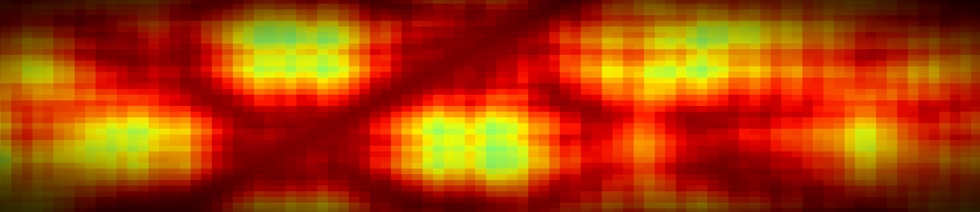ClassesComputational Classes

Members of the CSC are dedicated to the cause of undergraduate and graduate training in simulation-based engineering and science, focusing on topics in Scientific Computing, Parallel Computing, and courses stressing scientific computation in various disciplines.

Specific courses with these goals include [U = undergraduate, G = graduate]:

• Math 3315 / CSE 3365 -- Scientific Computing [U]
• Math 3316 -- High-Performance Scientific Computing [U]
• Math 4370 -- Parallel Scientific Computing [U]
• Math 5315 -- Numerical Analysis [U/G]
• Math 5316 -- Matrix Computation [U/G]
• Math 6315 -- Numerical Solution of Partial Differential Equations [G]
• Math 6316 / CSE 7366 -- Numerical Linear Algebra [G]
• Math 6320 -- Iterative Methods [G]
• Math 6321 -- Numerical Solution of Ordinary Differential Equations [G]
• Math 6360 -- Computational Electromagnetics [G]
• Math 6370 -- Parallel Scientific Computing [G]

Introduction to Computational Science

1. Compiled language programming in the Unix environment - (e.g. C, C++, F90).
2. Direct and iterative linear solvers with an introduction to relevant software (LAPACK).
3. Nonlinear solvers (Newton's method and variants, optimization).
4. Quadrature and differential equations with an introduction to relevant software.
5. Monte Carlo methods.

Introduction to Parallel Scientific Computing

1. Shared- and distributed-memory parallel programming and performance evaluation (e.g. OpenMP, MPI).
2. Parallel solver libraries (e.g. PETSc, ScaLAPACK, TriLinos, FFTW).
3. Applications to solving partial differential equations and/or large scale optimization and data analysis problems.